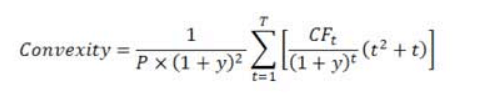#Bond Convexity Calculator

This online calculator is used to measure and manage the portfolios exposure to interest rate risk and risk of loss of expectation.

# Results...

Convexity = 26.26

Macaulay Duration = 4.82

Current Bond Price = \$911.37

Summary :

Bond of Face Value \$1000 with a Semi-Annual coupon of 8% and a yield of 10% and 6 years to maturity and a present price of 911.37 , the duration is 4.82 years and the Convexity is 26.26## Formula for Bond Convexity Calculation :

Convexity is a measure of the curve in the relationship between a bonds price and a bonds yield, as it also takes into account the bonds duration. The duration measures the sensitivity of an asset in relation to external market forces, such as interest rates.Where,

P - Bond Price

Y - Yield to maturity in decimal form.

T - Maturity in years.

CFt - Cash flow at time t.